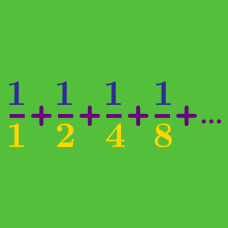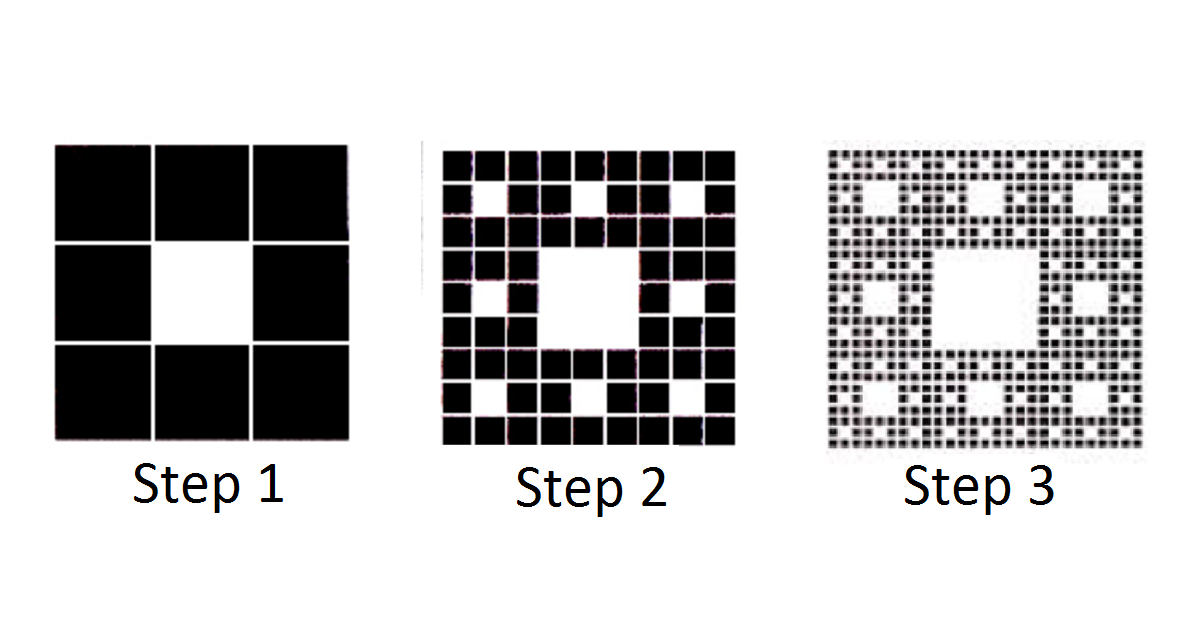Calculus

# Calculus Introduction 4

True or False?

For every quadratic function $f(x) = ax^2 + bx + c,$ there is a real number $x$ such that $f(x)=f'(x).$The Sierpinski carpet is formed in steps. First, a square with area 1 is divided into nine equally sized squares, and the middle square is removed. In each subsequent step, each remaining square is divided into nine equally sized squares and the middle square is removed.

What is the sum of the areas of the removed squares if this process continues infinitely?

Evaluate $\int_{0}^{3} \lfloor x \rfloor dx.$

Notation.$\lfloor x \rfloor$ represents the floor, or integer portion of $x.$ For example, $\lfloor 5.81 \rfloor = 5.$

For what positive integer $n$ is $\int_0^n \lfloor x \rfloor dx = \lfloor \int_0^n x dx \rfloor ?$

Notation.$\lfloor x \rfloor$ represents the floor, or integer portion of $x.$ For example, $\lfloor 5.81 \rfloor = 5.$

True or False?

For every $a > 2,$

$\sum_{n=0}^{\infty}\frac{1}{a^n} < \sum_{n=0}^{\infty}\frac{1}{n!}.$

Hint. $\sum_{n=0}^{\infty}\frac{1}{n!} = e.$

×